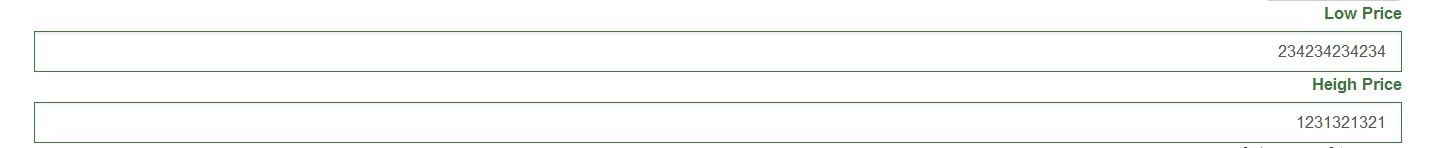# javascript error in yii.validation.php to perform your compar rules

when you want add a rules in your model and after that you want compare two input to see the which on is greater than an other you should add the compare for example:you have low_price and high_price as field in your model you add this codes in your model file:

``````
public function rules()

{

return [

[['low_price', 'heigh_price', 'one_price', 'project_id'], 'integer'],

[['project_id'], 'required'],

[['heigh_price'], 'compare', 'compareAttribute' => 'low_price', 'operator' => '>'],

];

}

``````

but in the view it is not work good as you can see in the picture .

6814when you change low_price to greater that high_price it is return true all two field but is should be false.for fix this error you should go to assest/849b9105/yii.validation.js

and find vlidations in at the 282 line as you can see at below

``````
switch (options.operator) {

case '==':

valid = value == compareValue;

break;

case '===':

valid = value === compareValue;

break;

case '!=':

valid = value != compareValue;

break;

case '!==':

valid = value !== compareValue;

break;

case '>':

valid = value > compareValue;

break;

case '>=':

valid = value >= compareValue;

break;

case '<':

valid = value < compareValue;

break;

case '<=':

valid = value <= compareValue;

break;

default:

valid = false;

break;

}

``````

and replace the valid = value > compareValue with if(value > compareValue){valid =true}else{valid =false}

as you can see in the below

``````
switch (options.operator) {

case '==':

valid = value == compareValue;

break;

case '===':

valid = value === compareValue;

break;

case '!=':

valid = value != compareValue;

break;

case '!==':

valid = value !== compareValue;

break;

case '>':

if(value > compareValue){valid =true}else{valid =false}

break;

case '>=':

valid = value >= compareValue;

break;

case '<':

valid = value < compareValue;

break;

case '<=':

valid = value <= compareValue;

break;

default:

valid = false;

break;

}

``````

you can fix another validation like that.

best regard

Are you sure?

``````

valid = value > compareValue

``````

is the same in effect with

``````

if(value > compareValue){

valid =true

} else {

valid =false

}

``````

I don’t believe it is the cause of your problem.

[EDIT]

Try this:

``````

[['heigh_price'], 'compare', 'compareAttribute' => 'low_price', 'operator' => '>', 'type' => 'number'],

``````

http://www.yiiframework.com/doc-2.0/yii-validators-comparevalidator.html#\$type-detail

The default value of ‘type’ is ‘string’. “234234” is greater than “123123123”.